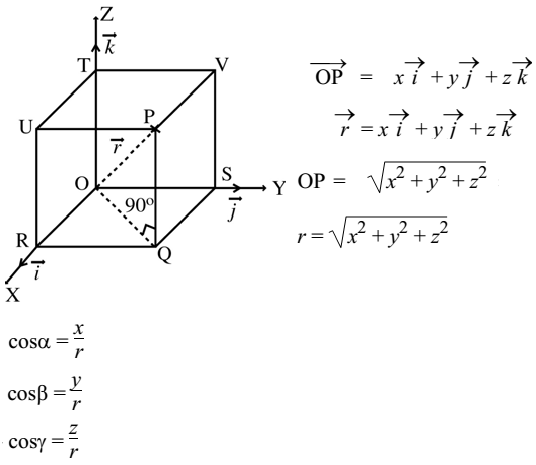# Direction Cosines

Let P(x, y, z) be any point in space with reference to a rectangular coordinate system O (XYZ). Let α, β and γ be the angles made by OP with the positive direction of coordinate axes OX, OY, OZ respectively. Then cos α, cos β, cos γ are called the direction cosines.Sum of the squares of direction cosines is unity.

cos2α + cos2β + cos2γ = 1

Sum of the squares of direction sines is 2.

sin2α + sin2β + sin2γ = 2